Homework:

1. Read Chapter 15 and complete Exercises 15.2, 15.3, 15.4, 15.7
2. Complete the Exercises given below.

I will ask for volunteers to present Exercises 1 and 2 (below) in class next lesson.  I will collect Exercise 3 on Thursday, November 10.

1. Prove that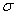is a multiplicative function by filling in the sketch of the proof provided below.

Theorem.  If gcd(m, n) = 1, then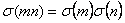.

Sketch of Proof.

a) Assume that m and n are two relatively prime positive integers, and prove that any divisor d of mn can be written uniquely as a product d1d2 where (d1, d2) = 1 and d1|m, d2|n.

b) Conversely, every pair of divisors d1|m, d2|n satisfying (d1, d2) = 1 gives rise to a divisor d1d2 of mn.

Hence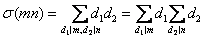.

2.   a) Prove that if f is a multiplicative function and n has prime factorization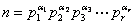, then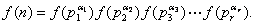b) Deduce that if n has prime factorization, then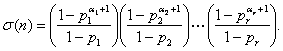3.   Work through parts a) through d) below to prove Euler’s Theorem.

Theorem (Euler).  Every even perfect number is of the form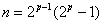where both p and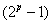are prime.

Proof.

a)      Suppose that n is an even perfect number and write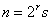, where s is odd.  Show that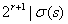and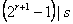.

b)      Let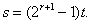Show that if t > 1, then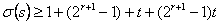; that is,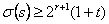.

c)      Derive a contradiction from the fact that t > 1; hence t = 1 and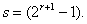Conclude that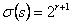.

d)      Explain why part c) implies that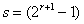prime.  Conclude that r +1 must be prime too.  Hence.